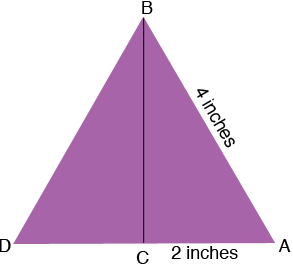SEARCH HOMEMath Central Quandaries & QueriesQuestion from anna, a student: I am anna and I am in 7th grade. i am trying to find the height of and equilateral triangle, all sides equaling 4 inchesHi Anna,

I drew a diagram of your triangle, labeled the vertices $A, B$ and $D,$ and drew a line from $B$ to $C,$ the mid point of $DA.$Triangle $CAB$ is a right triangle and hence you can use Pythagoras Theorem to find the length of $BC,$ the height of triangle $ABD,$

PennyMath Central is supported by the University of Regina and the Imperial Oil Foundation.